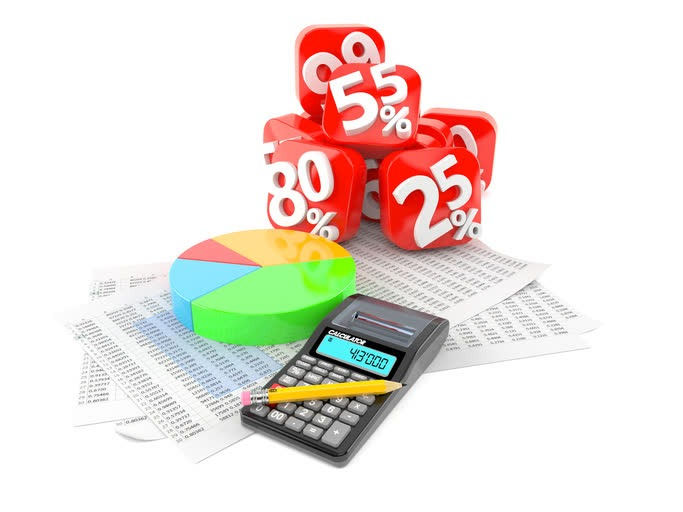Select Page

# Earnings Yield & How to Calculate Earnings YieldEarnings Yield, in its simplest form, is earnings divided by price. It is expressed as a percentage of the investment value and is the reciprocal of the price/earnings (PE ratio).

In other words, earnings yield is the annual earnings of a stock, individual company, or market index compared to the price.

Earnings Yield = Annual Earnings Per Share / Stock Price

or

Earnings Yield = Net Income / Market Capitalization

(Note that these calculations will provide the same result.)

## Examples of the Earnings Yield Calculation

Earnings Yield of a Stock
Apple (AAPL) earns \$9.20 per share, current price is \$171
Earnings Yield = \$9.20 / \$171 = 5.4%

Earnings Yield of a Company
Apple earns \$48.4 billion, Market Capitalization is \$896 billion.
Earnings Yield = \$48.4 billion / 896 billion = 5.4%

Earnings Yield of an Index
The S&P 500 earns \$105, the index is \$2625.
Earnings Yield = \$105 / 2625 = 4.0%

## Earnings Yield is the Reciprocal of the PE Ratio

P/E Ratio = Price / Earnings

Earnings Yield = Earnings / Price

The advantage of the earnings yield over the P/E Ratio is that your result is expressed as a percentage that can be compared to other investments.

For example:
S&P 500 earns 105, the index is \$2625 = 4.0%

compared to the

10 Year Treasury Bond Yield = 2.4%

The earnings yields gives you an easy to understand metric to compare versus using the P/E ratio. It’s easier to compare 4.0% earnings yield and 2.4% bond yield instead of a 25 P/E ratio versus a 2.4% bond yield. They both provide the same answer, but earnings yield provides a clearer comparison.

## Importance of the Earnings Yield

Earnings yield is both a rate of return metric and a valuation metric. The earnings are a key measurement of return. The fact it is divided by the current value also makes it a valuation metric.

Many investors put emphasis on dividends and dividend yield. But where do these dividends come from? Earnings of course. Earnings are the true long term driver of dividends and stock price.

You may have a stock that has a high dividend yield but pays out all of its earnings. This company may not have the capital to grow earnings or even sustain the high dividend. Earnings are one of the most important metrics in analyzing individual investments.

All of the above methods of calculating earnings to price yield provide a means to compare stocks, companies, indexes, etc. But this is also a valuable metric for comparing to other assets.

For instance, analysts compare the S&P500 earnings yield to the 10 year Treasury Bond yield (see example above), or to the current inflation rate. Treasury bills are considered the risk free rate of return. By comparing the earnings yield to Treasury bill rates you learn the “risk premium” of owning an equity versus a risk-free asset.

Use the earnings yield as a return and value metric because it’s simple and provides critical information that can be compared to any other investment asset.